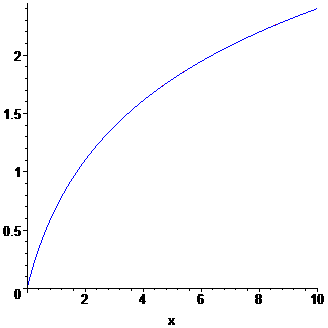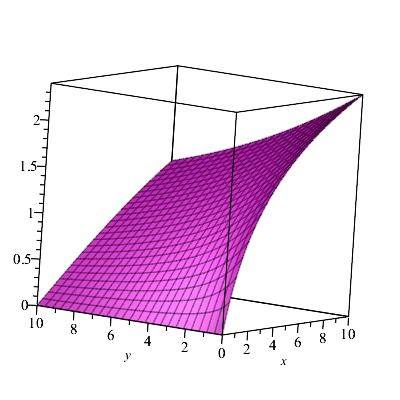# A solution to the Laplace equation

Moved from technical math section, so missing the homework template
Hi,
I am looking for the solution to the quadrant problem of the Laplace equation in 2 dimensions with Dirichlet boundary conditions
\begin{equation}
\frac{\partial^2 f}{\partial x^2} + \frac{\partial^2 f}{\partial y^2} = 0
\end{equation}
in the first quadrant ## x, y \geq 0 ## with boundary conditions ## f = \ln(1+x)## at ##y=0## and ##f=0## at ##x=0##.

I have looked at the solution in the book "Handbook of linear partial differential equations for engineers and scientists, 2nd edition, p. 800" but doing the integration with online algebra software (Mathematica, Maxima) gives nonsensical results that do not satisfy the boundary conditions.

Can you help me out with the solution?

Mark44
Mentor
Please show us what you have done so far. Since you are using computer algebra software, a screen shot would be helpful.

From the book, the general solution is
\begin{equation}
f(x,y) = \frac{4}{\pi} x y \int_0^{\infty} \frac{g_1 (\eta) \eta \, \mathrm{d} \eta}{\left[ (y-\eta)^2 + x^2 \right] \left[ (y+\eta)^2 + x^2 \right]} + \frac{4}{\pi} x y \int_0^{\infty} \frac{g_2 (k) k \, \mathrm{d} k}{\left[ (x-k)^2 + y^2 \right] \left[ (x+k)^2 + y^2 \right]}
\end{equation}
where ##f = g_1(y)## at ##x=0## and ##f=g_2(x)## at ##y=0##. In my case, ## g_1 = 0## and ## g_2(x) = \ln(1+x)##.

I don't know how to insert screenshots, so here is the Latex code of the indefinite integral (the definite integral could not be computed)
\begin{eqnarray}
\dfrac{1}{8xy} \mathrm{i}\left(-\ln\left(-\mathrm{i}y-x+1\right)\ln\left(\left|k+\mathrm{i}y+x\right|\right)+\ln\left(-\mathrm{i}y+x+1\right)\ln\left(\left|k+\mathrm{i}y-x\right|\right)+\ln\left(\mathrm{i}y-x+1\right)\ln\left(\left|k-\mathrm{i}y+x\right|\right)-\ln\left(\mathrm{i}y+x+1\right)\ln\left(\left|k-\mathrm{i}y-x\right|\right)+\operatorname{Li}_2\left(\frac{k+\mathrm{i}y+x}{\mathrm{i}y+x-1}\right)-\operatorname{Li}_2\left(\frac{k+\mathrm{i}y-x}{\mathrm{i}y-x-1}\right)-\operatorname{Li}_2\left(-\frac{k-\mathrm{i}y+x}{\mathrm{i}y-x+1}\right)+\operatorname{Li}_2\left(-\frac{k-\mathrm{i}y-x}{\mathrm{i}y+x+1}\right)\right)
\end{eqnarray}
Another form of this result apparently is
\begin{eqnarray}
-\dfrac{1}{8xy} \left(\operatorname{arctan2}\left(\frac{y\left(k+1\right)}{y^2+\left(x-1\right)^2}, -\frac{\left(x-1\right)\left(k+1\right)}{y^2+\left(x-1\right)^2}\right)\ln\left(\left(k+x\right)^2+y^2\right)-\operatorname{arctan2}\left(\frac{y\left(k+1\right)}{y^2+\left(x+1\right)^2}, \frac{\left(x+1\right)\left(k+1\right)}{y^2+\left(x+1\right)^2}\right)\ln\left(\left(k-x\right)^2+y^2\right)+\operatorname{arctan2}\left(k-x, y\right)\ln\left(\frac{\left(k+1\right)^2}{y^2+\left(x+1\right)^2}\right)-\operatorname{arctan2}\left(k+x, y\right)\ln\left(\frac{\left(k+1\right)^2}{y^2+\left(x-1\right)^2}\right)+\mathrm{i}\left(\operatorname{Li}_2\left(\frac{\mathrm{i}\left(k+x\right)+y}{y+\mathrm{i}\left(x-1\right)}\right)-\operatorname{Li}_2\left(-\frac{\mathrm{i}\left(k+x\right)-y}{y-\mathrm{i}\left(x-1\right)}\right)-\operatorname{Li}_2\left(\frac{\mathrm{i}\left(k-x\right)+y}{y-\mathrm{i}\left(x+1\right)}\right)+\operatorname{Li}_2\left(-\frac{\mathrm{i}\left(k-x\right)-y}{y+\mathrm{i}\left(x+1\right)}\right)\right)+2\ln\left(k+1\right)\left(\arctan\left(\frac{k+x}{y}\right)-\arctan\left(\frac{k-x}{y}\right)\right) \right)
\end{eqnarray}
This was done using an online integral calculator based on Maxima. Wolfram Alpha gives similar results.

What I find suprising is that setting ##y=0## cancels all the terms in the first representation of the integral even without considering the limits of integration and in the second case the boundary condition is not recovered either.

Ray Vickson
Homework Helper
Dearly Missed
From the book, the general solution is
\begin{equation}
f(x,y) = \frac{4}{\pi} x y \int_0^{\infty} \frac{g_1 (\eta) \eta \, \mathrm{d} \eta}{\left[ (y-\eta)^2 + x^2 \right] \left[ (y+\eta)^2 + x^2 \right]} + \frac{4}{\pi} x y \int_0^{\infty} \frac{g_2 (k) k \, \mathrm{d} k}{\left[ (x-k)^2 + y^2 \right] \left[ (x+k)^2 + y^2 \right]}
\end{equation}
where ##f = g_1(y)## at ##x=0## and ##f=g_2(x)## at ##y=0##. In my case, ## g_1 = 0## and ## g_2(x) = \ln(1+x)##.

I don't know how to insert screenshots, so here is the Latex code of the indefinite integral (the definite integral could not be computed)
\begin{eqnarray}
\dfrac{1}{8xy} \mathrm{i}\left(-\ln\left(-\mathrm{i}y-x+1\right)\ln\left(\left|k+\mathrm{i}y+x\right|\right)+\ln\left(-\mathrm{i}y+x+1\right)\ln\left(\left|k+\mathrm{i}y-x\right|\right)+\ln\left(\mathrm{i}y-x+1\right)\ln\left(\left|k-\mathrm{i}y+x\right|\right)-\ln\left(\mathrm{i}y+x+1\right)\ln\left(\left|k-\mathrm{i}y-x\right|\right)+\operatorname{Li}_2\left(\frac{k+\mathrm{i}y+x}{\mathrm{i}y+x-1}\right)-\operatorname{Li}_2\left(\frac{k+\mathrm{i}y-x}{\mathrm{i}y-x-1}\right)-\operatorname{Li}_2\left(-\frac{k-\mathrm{i}y+x}{\mathrm{i}y-x+1}\right)+\operatorname{Li}_2\left(-\frac{k-\mathrm{i}y-x}{\mathrm{i}y+x+1}\right)\right)
\end{eqnarray}
Another form of this result apparently is
\begin{eqnarray}
-\dfrac{1}{8xy} \left(\operatorname{arctan2}\left(\frac{y\left(k+1\right)}{y^2+\left(x-1\right)^2}, -\frac{\left(x-1\right)\left(k+1\right)}{y^2+\left(x-1\right)^2}\right)\ln\left(\left(k+x\right)^2+y^2\right)-\operatorname{arctan2}\left(\frac{y\left(k+1\right)}{y^2+\left(x+1\right)^2}, \frac{\left(x+1\right)\left(k+1\right)}{y^2+\left(x+1\right)^2}\right)\ln\left(\left(k-x\right)^2+y^2\right)+\operatorname{arctan2}\left(k-x, y\right)\ln\left(\frac{\left(k+1\right)^2}{y^2+\left(x+1\right)^2}\right)-\operatorname{arctan2}\left(k+x, y\right)\ln\left(\frac{\left(k+1\right)^2}{y^2+\left(x-1\right)^2}\right)+\mathrm{i}\left(\operatorname{Li}_2\left(\frac{\mathrm{i}\left(k+x\right)+y}{y+\mathrm{i}\left(x-1\right)}\right)-\operatorname{Li}_2\left(-\frac{\mathrm{i}\left(k+x\right)-y}{y-\mathrm{i}\left(x-1\right)}\right)-\operatorname{Li}_2\left(\frac{\mathrm{i}\left(k-x\right)+y}{y-\mathrm{i}\left(x+1\right)}\right)+\operatorname{Li}_2\left(-\frac{\mathrm{i}\left(k-x\right)-y}{y+\mathrm{i}\left(x+1\right)}\right)\right)+2\ln\left(k+1\right)\left(\arctan\left(\frac{k+x}{y}\right)-\arctan\left(\frac{k-x}{y}\right)\right) \right)
\end{eqnarray}
This was done using an online integral calculator based on Maxima. Wolfram Alpha gives similar results.

What I find suprising is that setting ##y=0## cancels all the terms in the first representation of the integral even without considering the limits of integration and in the second case the boundary condition is not recovered either.

Your solution does seem to satisfy the boundary condition for ##y=0##. Using a small non-zero value ##y = 0.001## we can plot the value of the solution
$$f(x,y) = \frac{4 x y}{\pi} \int_0^{\infty} \frac{u \ln(1+u)}{[(x-u)^2+y^2)] [(x+u)^2+y^2]}$$
as a function of ##x## and compare it with the graph of ##\ln(1+x)##. I did this using Maple, and the results are given below. I just left the integral unevaluated and let Maple compute it numerically, but the same result was obtained if Maple did it symbolically.

The two graphs ##f(x,0.001)## in blue and ##\log(1+x)## in red coincide, and just look like a single blue plot. I also (separately) plotted ##f(x,0.001)_{\text{numerical}}## and ##f(x,0.001)_{\text{analytical}}## together, and they likewise coincide.#### Attachments

•Delta2
Ray Vickson
Homework Helper
Dearly Missed
Hi,
I am looking for the solution to the quadrant problem of the Laplace equation in 2 dimensions with Dirichlet boundary conditions
\begin{equation}
\frac{\partial^2 f}{\partial x^2} + \frac{\partial^2 f}{\partial y^2} = 0
\end{equation}
in the first quadrant ## x, y \geq 0 ## with boundary conditions ## f = \ln(1+x)## at ##y=0## and ##f=0## at ##x=0##.

I have looked at the solution in the book "Handbook of linear partial differential equations for engineers and scientists, 2nd edition, p. 800" but doing the integration with online algebra software (Mathematica, Maxima) gives nonsensical results that do not satisfy the boundary conditions.

Can you help me out with the solution?

Here is a 3d-plot of the solution
$$f(x,y) = \frac{4 x y}{\pi} \int_0^{\infty} \frac{u \ln(1+u)}{[(x-u)^2+y^2)] [(x+u)^2+y^2]}\, du,$$
as done using Maple. In plotting, if the integral is "tricky" to do analytically, Maple will just evaluate it numerically.